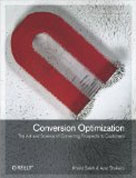• # Bayesian A/B Testing vs Frequentist A/B Testing – What’s the Difference?### Bayesian versus Frequentist Statisticians: the war is real

Imagine that you wake up in the one morning and you don’t remember anything from your previous life. You’ve erased all memories from your previous days.

Pretty intense and terrifying, huh?

Well, that’s how Bayesian statisticians describe frequentist colleagues because frequentist statisticians do not use any prior knowledge. Everything you learn about the world is through the lens of events that are happening at the moment and currently.

Michael Hochster explains;

“The essential difference between Bayesian and Frequentist statisticians is in how probability is used.”

On the contrary, the anti-Bayesian position is described well in this viral joke;

“A Bayesian is one who, vaguely expecting a horse, and catching a glimpse of a donkey, strongly believes he has seen a mule.”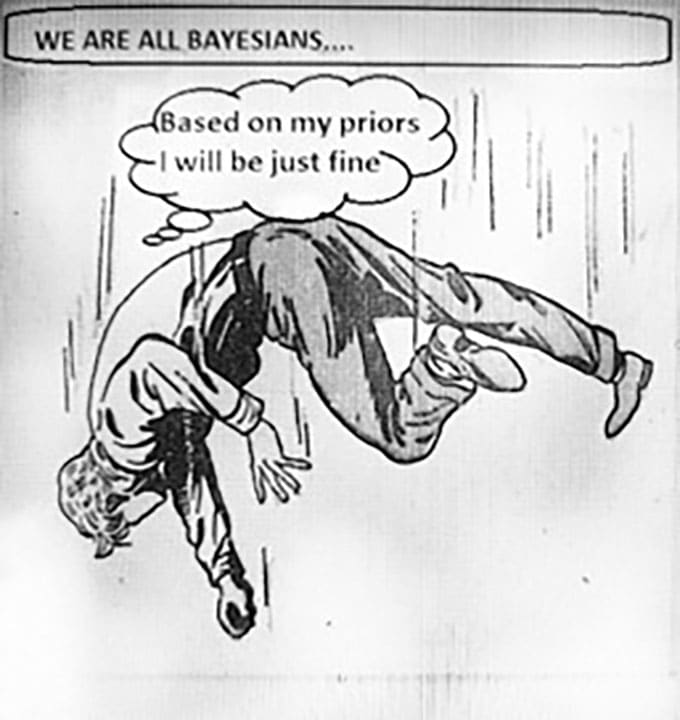What does this have to do with statistics and why is it important?

If you’re doing any AB testing this is relevant to you. To sum it up: as a Bayesian statistician, you use your prior knowledge from the previous experiments and try to incorporate this information into your current data. As a Frequentist statistician, you are using only data from your current experiment. Moreover, when formulating any conclusions, i.e. formulating so-called statistical inference, frequentist methods assume that you repeat your experiment many, many times.

The Cthaeh explains;

“The difference between frequentist and Bayesian approaches has its roots in the different ways the two define the concept of probability. Frequentist statistics only treats random events probabilistically and doesn’t quantify the uncertainty in fixed but unknown values (such as the uncertainty in the true values of parameters). Bayesian statistics, on the other hand, defines probability distributions over possible values of a parameter which can then be used for other purposes.”

### Example:

Let’s say, you run an e-commerce website and you are tasked with increasing the conversion rate for visitors who come to the cart page. Your existing cart page receives 10,000 visitors per month and generates 5,000 conversions. So the conversion rate for the cart page is 5,000/10,000 * 100 = 50%

You create a new design for the cart page (design B) and you want to test if design B generates higher conversion rate compared to the control conversion

To do that, you decide to run an AB test between the control (design A) and the challenger (design B).  While running the test, you observe that the control is reporting a 60% conversion rate. The A/B testing software reports conversion rates for the challenger as well.

### Frequentist solution: conduct a test

As a frequentist, you first formulate the hypothesis of interest which is called a null hypothesis and it states:

“a conversion rate for A   is equal to a conversion rate for B  “

It is important to understand that when you are running an AB test, you are analyzing the behavior of a sample from the population. The population, in this case, all the visitors who will come to the page at any point in time. The sample is the visitors who go through the test. This sample is limited to the time when are running the test. Your goal is to analyze the behavior of that sample and predict how the general population will react based on that sample. Going back to our cart page example, let’s say that the cart page gets 10,000 visitors per month. We will run our test for one month. So our sample size is 10,000 visitors. What is the population size? The population is all visitors who will come to the cart as long as the site is running. The cart page might get hundreds of thousands of visitors. So, is the behavior of the 10,000 visitors who came to the cart page and saw either the control or the new design enough to predict how hundreds of thousands of visitors will react to these designs?You never know the truth.

As you are running the test for a small sample of the overall population and you don’t observe the true conversion rate for all the user’s population. An A/B test gives you an estimate of the sample taken from that population.

So, how do you know that your sample population will provide a correct estimate of how the overall population will react?

#### Consider scenario 1:

1. Based on your sample population and AB test results, you conclude that the challenger will increase conversion rates (so you reject the null hypothesis of both rates)
2. In reality (for the whole population of visitors), the challenger will NOT increase conversion rates for the overall population

In this case, based on your test data for the sample population, you REJECTED a TRUE hypothesis. This is called a type I error  (false positive).

#### Consider scenario 2:

1. Based on your sample population and AB test results, you conclude that the challenger will not increase conversion rates (so you do not have enough evidence to reject the null hypothesis about the equality of both rates)
2. In reality, the challenger will increase conversion rates for the overall population

In this case, based on your test data, you did NOT REJECT a FALSE hypothesis. This is called a type II error (false negative).How do you make sure you do not fall into this trap? You construct the test in such a way to keep the probability of scenario 1 (wrongly rejecting the true hypothesis) at the very small amount which is usually assumed to be 0.05 (so-called significance level). You will also need to construct your test to minimize the probability of scenario 2 (not rejecting the hypothesis that should be rejected when it’s false). The probability of this type of mistake is controlled by your sample size, i.e. you traffic that goes through the test. The more sample, the more probability of rejecting of the false hypothesis (more power) and stating that the conversion rate for A is less or greater the conversion rate for B.

### Bayesian solution: data + prior belief = conclusion

On the other hand, as a Bayesian statistician, you have not only the data, i.e. a current conversion rate of 60% for A and a current rate for B.  You also have the prior knowledge about the conversion rate for A which for example you think is closer to 50% based on the historical data. It means that when running a split test, you observe the control 60% conversion rate, but you know that historically, it is typically at 50%.

Usually, you do not have the same knowledge of the conversion rate for the challenger (design B) since it is new, not tested before. If you really believe that the conversion rate for the control is overestimated (it should be lower) in your current experiment, as compared to the previous experiments, you can use so-called strong prior distribution around 0.5 for this particular parameter. By strong prior I mean something like below: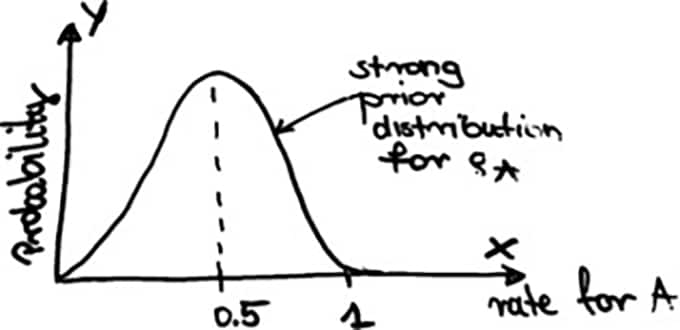On this graph, you see how your prior belief looks like in a sense that you put a high probability on the value that you believe in and much less probability around all the other values.

On the other hand, if you are not so sure about the 0.5, you can use a weaker prior to the following: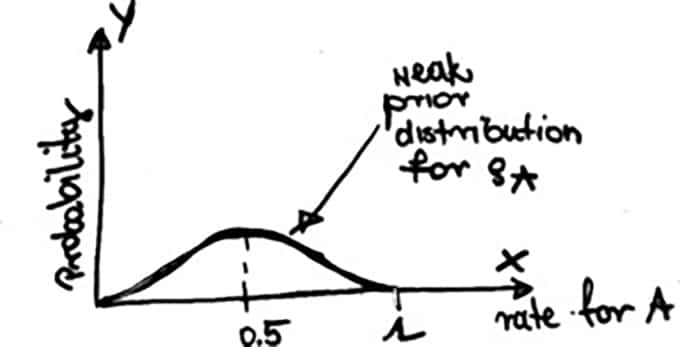And in the extreme case, when you do not have any prior knowledge about the conversion rate for A (ρa), you just put the same probability for all possible values like below: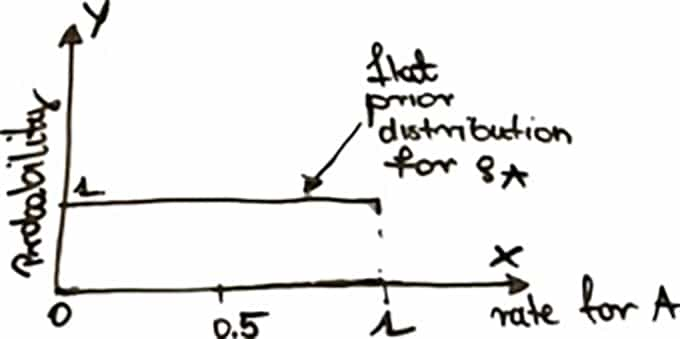Here the probability for ρa is 1 for all possible values and this is because the area under the density function must be 1 in total as for all statistical distributions. This case of prior is called a flat prior or non – informative or vague prior. In fact, you do not incorporate any prior knowledge here.

In the Bayesian approach, you must specify a prior also for a rate B, even if you do not have any prior knowledge of it. Usually, you use the weak prior then.

Let’s say you chose a strong prior for A and a weak for B. What next? The second component of the Bayesian inference is a so-called likelihood. As a Bayesian, you do not just use only a point estimate for ρaand ρ(like the frequentist does) but you construct so-called likelihood function which is the function of the parameter of a statistical model given your data. It is completely based on your data. To be more specific, a likelihood is a function of your data. Let’s say you have n visitors and n results for them. Then, the likelihood function is telling you what is the probability of what you have just observed for all those users, giving that the true conversion rate for A and B are known. Then the likelihood function would be a surface since it has 2 arguments: rate for A and rate for B. Let’s assume that you observed 80% rate for B in your current experiment. The maximum value of the likelihood (peak of the surface) would be at the observed rate of 60% and 80%.  This is what you have seen from your current data. 80% and 60% are therefore the most probable values for the conversion rate for A based and B just on your data.

Okay but what about the shapes of all these distributions?

Are they coming from the data too? No, you choose the shape of a prior and the likelihood function distribution. You must assume it. And of course, you need to choose one of the known statistical distributions such as normal, Bernoulli, etc. It requires some statistical knowledge or an expert help.

Okay so what next? I have the priors and the likelihood. How to combine them?

This is the moment when the Bayes theorem comes into the play and helps you to obtain a result which is called the posterior distribution. Posterior is a conditional probability function, ie. the probability that the two conversion rates (for a control and a challenger) will be some vector (two values together) ρ =(ρA, pB)  given the data from your current experiment.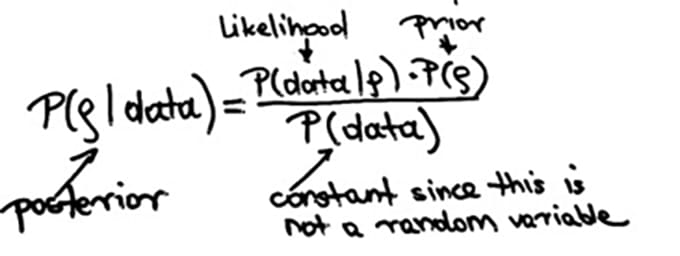Since P(data) is a constant (data is not a random variable), the posterior distribution is proportional to the likelihood times the prior. By the prior we mean again a vector, so the two priors pair, for A and B together.

## How is the posterior computed?

Sometimes the posterior is known, i.e. the shape and parameters can be derived easily from the mathematical theory. And if it is not the case you must rely on some computational algorithms.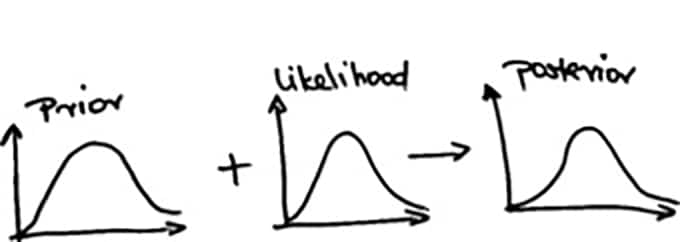### How to make conclusions based on the posterior distribution?

You just look at it, once you have it, and you see whether your prior expectations were right or wrong. Is the posterior for A concentrated around 0.5 value as expected or not? For more formal inference you might construct the whole interval of all most probable values for both rates A and B, based on the obtained posterior distribution (so-called credibility interval).  It is analogical to the confidence interval in frequentist approach, but it has different mathematical interpretation. It comes from the fact that frequentists consider rate parameters to be fixed and data to be random, while Bayesians consider rate parameters to be random and data to be fixed. Let’s say we constructed the interval for the difference between the two rates A and B.  Both intervals are numerically equivalent but their interpretation is as follows.

“Given our observed data, there is a 95% probability that the true value of the difference between the two rates falls within the interval” – Bayesians.

“There is a 95% probability that when I compute the difference between the two rates in any experiment of this type, the true difference will fall within the interval” – Frequentists.

Because, in a frequentist approach, you have a problem with multiple testing. The more tests you make, the higher the probability that you obtain AT LEAST ONE false significant result (you reject the true hypothesis). It considers comparing one control A to a new variation B and the same control A to C or comparing A with B, A with C and B with C. No matter what, in the frequentists approach the probability that you obtain at least one significant result increases incredibly. This comes from the fact that in every single test there is always a probability that you can make a type I error mistake, i.e. the probability of rejecting the true hypothesis. The probability of making at least one such a mistake increases very fast when you make more comparisons, according to the mathematical formula which we omit here. It is illustrated by the graph below (the flat dotted line is for the error of 0.05). It’s like flipping a coin many times waiting for the heads. The more you try, the more probability you get it.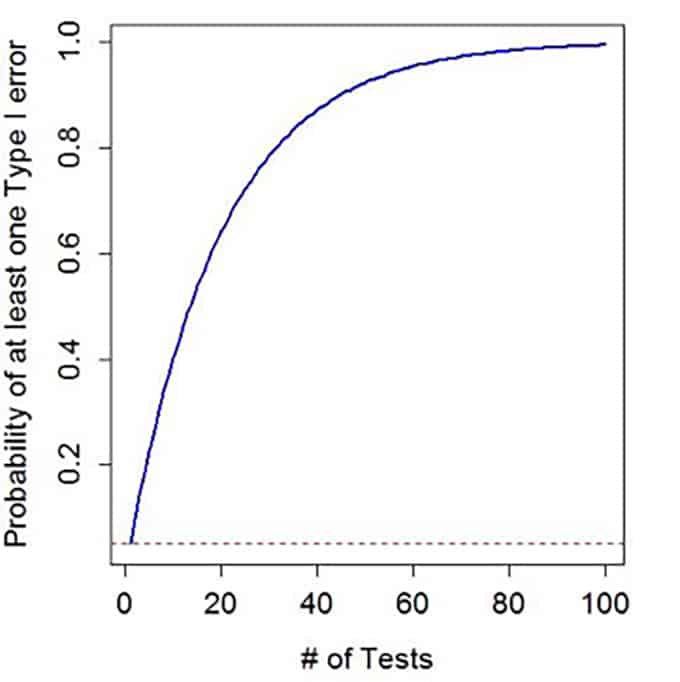There are of course some so-called “corrections” to the multiple testing problems like Bonferroni or Hochberg but they require more statistical knowledge plus you must decide which one to choose. Some are more and some less conservative.

This problem disappears if you opt for Bayesian methods.

Should we be afraid of strong priors?

Even though the main feature in Bayesian approach is a prior belief when it comes to a practical application one of the most often choices of the prior distribution is vague prior that you have seen before. People often choose non-informative priors because they know that too strong prior can dominate the posterior and they are afraid of it. In fact, if you have a very strong prior belief you don’t need any data to tell you something new. That’s why a non-informative prior is good choice to start with and after that, as the experiment goes, you can modify it, once you get some knowledge. You can treat your posterior distribution as a new prior to the next experiment. Therefore, each time you update your prior using the new data. For our 2 priors example, it may go as follows:

First two priors for A and B (strong +non-informative) +data from experiment 1

→First Posterior for A (A1) +Posterior for B (B1)

Second priors (Posterior A1 and Posterior B1)                   +data from experiment 2

→Second Posterior for A (A2)+ Posterior for B (B2)

n-th prior for A  (Posterior A n-1) + n-th prior for B (Posterior n-1)

+data from experiment n → n-th Posterior for A (An) +Posterior for B (Bn)

So you can use a first strong prior for A and a weak one for B.  Then, you can use posterior distributions for the conversion rate for A and B concentrated around the obtained value as the next priors in another experiment and so on.

Who is the father of the Bayesian statistics? A bit of history.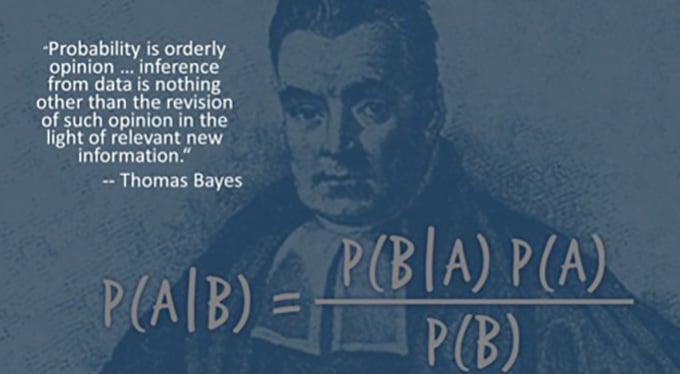In 1763 a work a paper called “An Essay towards solving a Problem in the Doctrine of Chances” written by an English statistician, Thomas Bayes, was presented, two years after his death. It contained his famous Bayes’ theorem you have seen before. Even though Bayes formulates his famous postulate in his paper, he didn’t interpret than the usage of the prior knowledge in a way the modern Bayesian statistician do it now. This took place much later. Around 1950, the Bayesian “big bang” took place thanks to the developments of the computing technology. It allowed the Bayes’ theory to be finally used in practical applications.

The frequentists methods, as based on the idea of drawing conclusions from the sample using the frequency or proportion of the data, are much older than the Bayesian ones. In fact, already the Athenians calculated the height of the wall of the wall of Platea by counting the number of bricks in a section of the wall and the procedure was repeated several times by a number of soldiers.

From this perspective, Bayesian methods are very fresh. Even after developing a Bayesian kind of perspective only the very limited number of practical applications were feasible to conduct (mainly on the paper). The very late popularity of Bayesian modeling was therefore caused merely not because people didn’t know how to use prior knowledge but because in most of the cases it was not possible to derive an exact solution to their problems and approximate solutions were not an option without the computer support.

Summary take-home messageWhatever choice you make be aware of the advantages and disadvantages of the frequentists and Bayesian methods. Once you use only vague priors, Bayesian method becomes just another estimation method, yet it protects you from multiple testing problems and allows for more flexibility. Of course, as they say, “there is no free lunch” and often you have to be prepared for computation burden when using Bayesian methods. The good news is that in case of A/B testing problem it is not necessary since you can rely on exact derivations when working with proper priors. Things get complicated if you want to change them.  Then you must be prepared for more computational difficulties and complexity. Finally, it is always a good idea to do so-called “sensitivity analysis”, i. e. to see how your prior choices impact the final results and conclusions.

### How should we choose a prior distribution?

Conjugate prior + data distribution: a perfect match.

A bit of mathematical knowledge will help you to choose the shape of your prior distribution that combines well with the distribution of your data. Statisticians call this perfect prior a conjugate prior. To be more specific, a prior is a conjugate if a posterior is the same functional form as the prior. In any case, a prior should reflect what you believe your current parameter is. It should be concentrated around the value that you obtained in your or someone else experiments. Roopam Upadhyay explains;

“Conjugate priors form a harmonic relationship with the distributions of data (evidence) to produce easy to decipher posterior distributions. This is similar to mixing distilled water from two different rivers and getting more distilled water which has the same properties as the prior distribution.  Non-conjugate priors, on the other hand, is like mixing soil and water. In this case, the posterior is like sticky mud which is hard to work with.”

### What if my prior is not conjugate?Then your computer will help you to derive not the exact posterior distribution but to sample from it. It is usually a very time-consuming process. The good news is that there are nowadays many statistical programs that do the job for you. The bad news is that they are usually helpful in a simple book-example case, not real-life problems. That means that you can wait hours and usually days or weeks in order the process to finish and even then, the results may not be reliable.

References

# Khalid Saleh

Khalid Saleh is CEO and co-founder of Invesp. He is the co-author of Amazon.com bestselling book: "Conversion Optimization: The Art and Science of Converting Visitors into Customers." Khalid is an in-demand speaker who has presented at such industry events as SMX, SES, PubCon, Emetrics, ACCM and DMA, among others.

View All Posts By Khalid Saleh## 2 thoughts on “Bayesian A/B Testing vs Frequentist A/B Testing – What’s the Difference?”

1.Job van Hardeveld says:

Well done Khalid, really interesting topic and post.

There is a small typo in the example by the way:

So the conversion rate for the cart page is 6.000/10.000 * 100 = 50%

2.Khalid Saleh says:

Thank you Job! Glad to hear that you enjoyed it. Took almost three weeks to write it.

I fixed the typo 🙂

•